# The mean-value conjectures

For convenience we state examples of the three main conjectures for moments in families. There are no open questions in this article (except how to prove these conjectures!). For simplicity, we state the conjectures only for integral moments.

Unitary

Let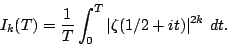Conjecture.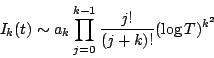where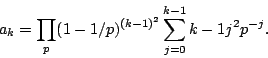Symplectic

Let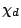be a real, primitive, quadratic character to the modulus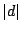(i.e. a Kronecker symbol) and let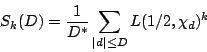where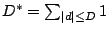.

Conjecture.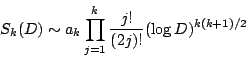where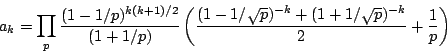Orthogonal

Let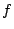be a normalized newform of weight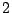and level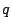(whereis prime) (we write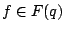and let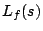be the associated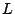-function (with critical strip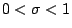.) Let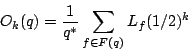where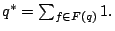Conjecture.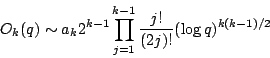where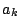is a product over primes (which can be worked out for any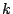, but for which we don't have a simple closed form expression);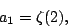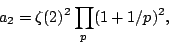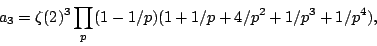and so on.

Back to the main index for L-functions and Random Matrix Theory.